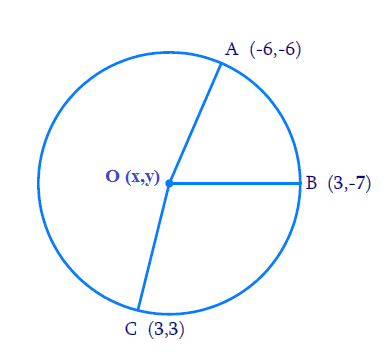# Ex.7.4 Q3 Coordinate Geometry Solution - NCERT Maths Class 10

Go back to  'Ex.7.4'

## Question

Find the centre of a circle passing through the points

$$\left( {6,- 6} \right), \left( {3, - 7} \right){\text{ and }} \left( {3,3} \right).$$

Video Solution
Coordinate Geometry
Ex 7.4 | Question 3

## Text Solution

Reasoning:

The distance between the two points can be measured using the Distance Formula which is given by:

Distance Formula

$$\text=\sqrt{\left(x_{1}-x_{2}\right)^{2}+\left(y_{1}-y_{2}\right)^{2}}$$

What is known?

The $$x$$ and $$y$$ co-ordinates of the points.

What is unknown?

The centre of the circle passing through the points

$$\left( {6,- 6} \right), \left( {3, - 7} \right){\text{ and }} \left( {3,3} \right).$$

Steps:

From the Figure,Given,

• Let \begin{align}O\left( {x,y} \right)\end{align} be the centre of the circle.
• Let the points \begin{align}\left( {6,\, - 6} \right),\,\,\left( {3, - 7} \right),\,\,\text{and}\left( {3,3} \right)\end{align} be representing the points $$A$$, $$B$$, and $$C$$ on the circumference of the circle.

Distance from centre $$O$$ to $$A$$, $$B$$, $$C$$ are found below using the Distance formula mentioned in the Reasoning.

\begin{align}∴ OA & = \sqrt {{{(x - 6)}^2} + {{(y + 6)}^2}} \\ OB &= \sqrt {{{(x - 3)}^2} + {{(y + 7)}^2}} \\ OC &= \sqrt {{{(x - 3)}^2} + {{(y - 3)}^2}} \end{align}

From the figure that

\begin{align}OA &= OB \\ ( \text{radii of} & \text{ the same circle} ) \end{align}

\begin{align} \sqrt {\! (x\! - \!6)^2\! +\! (y \! +\! 6)^2 } & \! = \! \sqrt {\! (x \! -\! 3)^2 \!\! + \! (y \! + \! 7)^2 } \\\begin{bmatrix} {x^2} + 36 - 12x +\\ {y^2} + 36 + 12y \end{bmatrix} &\!=\! \begin{bmatrix} {x^2} + 9 - 6x +\\ {y^2} + 49 + 14y \end{bmatrix} \\\\\text{(Squaring on } & \text{both sides)}\\\\- 6x - 2y + 14 &= 0 \\ 3x + y &= 7 \ldots \text{Equation} (1) \end{align}

Similarly,

\begin{align}OA & = OC \\ ( \text{radii of} & \, \text{ the same circle} ) \end{align}

\begin{align} \! \sqrt { \! (x \! - \! 6)^2 \! + \! (y \! +\! 6)^2 } & \! = \! \sqrt {\! (x \! - \! 3)^2 \!+\! (y\! - \! 3)^2 }\\\begin{bmatrix} {x^2} + 36 - 12x + \\{y^2} + 36 + 12y \end{bmatrix} &= \begin{bmatrix} {x^2} + 9 - 6x + \\{y^2} + 9 - 6y \end{bmatrix}\\\\\text{(Squaring on } &\text{both sides)}\\\\- 6x + 18y + 54 &= 0 \\ - 3x + 9y &= - 27 \ldots(2) \end{align}

On adding Equation $$(1)$$ and Equation $$(2)$$, we obtain

\begin{align}10y &= - 20\\y &= - 2\end{align}

From Equation $$(1)$$,we obtain

\begin{align}3x - 2{\text{ }} = {\text{ }}7\\3x = {\text{ }}9\\x = {\text{ }}3\end{align}

Therefore, the centre of the circle is $$(3, -2)$$.

Learn from the best math teachers and top your exams

• Live one on one classroom and doubt clearing
• Practice worksheets in and after class for conceptual clarity
• Personalized curriculum to keep up with school